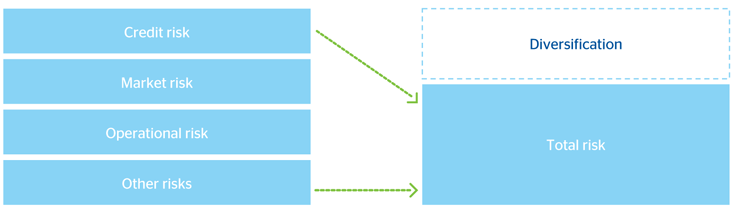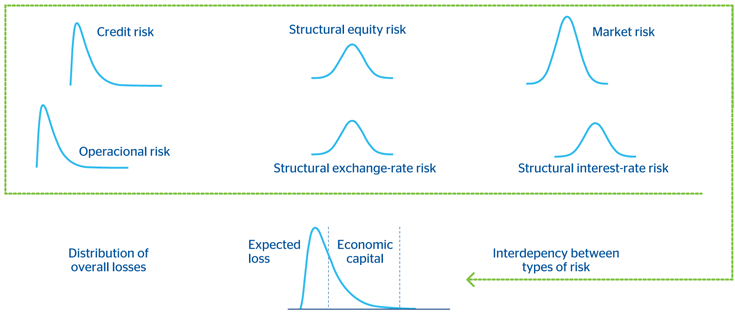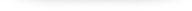# Integration of risks

The economic capital required to cover the Group’s losses is calculated by integrating the various risks managed by the Entity. The difference between this economic capital and the sum of the individual capital amounts is known as the benefit of diversification.

###### Sum of risks and diversificationBBVA Group’s risk integration model recognizes diversification among the various types of risks. The calculation process is divided into two stages. In the first stage, each of the risks is modeled individually (credit, market, structural and operational), taking the special features of each case into account. In the second stage, they are added to a common measure through a model that looks at the structure of dependency between risks.

###### BBVA Group. Risk integration diagramIn this framework, the diversification level of each risk depends mainly on the relative size of the risk against global risk, as well as on the correlation among different risks and the characteristics of individual loss distributions.

Tools